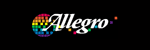电子元器件数据表 IC PDF查询English中文版 品牌 我要上传
 型号： 开始 精确 包含 结束 描述：
A1322ELHLT-T 比例式线性霍尔效应传感器的高温作业 (Ratiometric Linear Hall Effect Sensor for High-Temperature Operation).型号：   A1322ELHLT-T
PDF文件： 下载PDF文件   鼠标右键选目标另存为

Ratiometric Linear Hall Effect Sensor for High-Temperature Operation

Logo：PDF原版 中文翻译版100%A1321, A1322,
A1323

J（下最大）
。下的某些组合

J
。 （热数据也可在

θJA

gure优点sum-
marizing应用程序的能力和在设备消散

θJC
，是

θJA
。周围空气温度，
T
A

D
） ，可以

J

D
.
P
D
= V
IN
×
I
IN
ΔT
= P
D
×
R
θJA
(2)
T
J
= T
A
+
ΔT
(3)
(1)

CC

A
= 150℃，封装UA ，使用

R
θJA
= 165 ° C / W ，T
J（下最大）
= 165 ° C，V
CC（ MAX）的
= 5.5 V ，和
I
CC（ MAX）的
= 8毫安。

D（最大）
。首先，

ΔT

= T
J（下最大）
– T
A
= 165 °C – 150 °C = 15 °C

J

P
D（最大）
=
ΔT

÷ R
θJA
= 15 °〜 165 ° C / W = 91毫瓦

V
CC （ EST ）
= P
D（最大）
÷ I
CC（ MAX）的
= 91毫瓦÷8 MA = 11.4 V

A

≤V
CC （ EST ）
.

CC （ EST ）

CC（ MAX）的
。如果V
CC （ EST ）
V
CC（ MAX）的
，那么可靠性
V之间能够操作
CC （ EST ）

CC（ MAX）的

R
θJA
。如果V
CC （ EST ）
V
CC（ MAX）的
，则V之间的合作
CC （ EST ）
V
CC（ MAX）的

A
= 25°C,
V
CC
= 12 V，I
CC
= 4毫安，而R
θJA
= 140℃ / W ，则：
P
D
= V
CC
×
I
CC
= 12 V
×
4毫安= 48毫瓦
ΔT
= P
D
×
R
θJA
= 48毫瓦
×
140 ° C / W = 7°C
T
J
= T
A
+
ΔT
= 25°C + 7°C = 32°C

D（最大）
，代表了最大允许

CC（ MAX）的
, I
CC（ MAX）的
） ，在不超过牛逼
J（下最大）
,

θJA

A
.
Allegro MicroSystems公司
115东北托夫，箱15036

www.allegromicro.com
9
 首页 - - 友情链接 Copyright© 2001 - 2014 ICPDF All Rights Reserved ICPDF.COM粤公网安备 44030402000629号粤ICP备13051289号-7